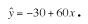### CFA Practice Question

There are 208 practice questions for this study session.

### CFA Practice Question

Forecasters use regression to predict the volume of timber in a tree using easily measured quantities such as diameter. Let y be the volume of timber in cubic feet and x be the diameter in feet (measured at 3 feet above ground level). One set of data gives:The predicted volume for a tree of diameter = 18 inches is:

A. 1050 cubic feet.
B. 90 cubic feet.
C. 60 cubic feet.

The prediction equation multiplies the value of x = 1.5 feet by 60 and then adds the intercept which is -30 cubic feet.